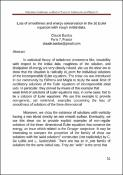## Loss of smoothness and energy conservation in the 3d Euler equation with rough initial data.

 dc.contributor.author Claude Bardos dc.date.accessioned 2017-05-03T09:37:01Z dc.date.available 2017-05-03T09:37:01Z dc.date.issued 2010-08-02 dc.description.abstract

In statistical theory of turbulence phenomena like, instability with respect to the initial data, roughness of the solution, and dissipation of energy are very closely related. We use the shear ow to show that the situation is radically di_erent for individual solutions of the incompressible Euler equations. The shear ow was introduced in our community by DiPerna and Majda to study the weak limit of oscillatory solutions of the Euler equations of incompressible ideal uids. In particular, they proved by means of this example that
weak limit of solutions of Euler equations may, in some cases, fail to be a solution of Euler equations. We use this example to provide non-generic, yet nontrivial, examples concerning the loss of smoothness of solutions of the three-dimensional

Moreover, we show the existence of solutions with vorticity having a non trivial density on non smooth surface. Eventually, we use this shear ow to provide explicit examples of non-regular solutions of the three- dimensional Euler equations that conserve the energy, an issue which related to the Onsager conjecture. It may be interesting to compare the properties of the family of shear ow solutions with the \wild solutions" constructed (not explicitely) by C. De Lellis and L. Szekelyhidi. There one has an in_nite family of solutions for the same initial data. They are "wild" in the sense that

they are limit on oscillating velocity _elds and they conserve the energy. In relation view an "optimistic" construction of a measure for statistical theory of turbulence one could imagine that in both cases one has family of solutions of measure zero. (In spite of the fact that in the second case the wild solutions form a residual set)

en dc.description.abstract

In statistical theory of turbulence phenomena like, instability with respect to the initial data, roughness of the solution, and dissipation of energy are very closely related. We use the shear ow to show that the situation is radically di_erent for individual solutions of the incompressible Euler equations. The shear ow was introduced in our community by DiPerna and Majda to study the weak limit of oscillatory solutions of the Euler equations of incompressible ideal uids. In particular, they proved by means of this example that
weak limit of solutions of Euler equations may, in some cases, fail to be a solution of Euler equations. We use this example to provide non-generic, yet nontrivial, examples concerning the loss of smoothness of solutions of the three-dimensional

Moreover, we show the existence of solutions with vorticity having a non trivial density on non smooth surface. Eventually, we use this shear ow to provide explicit examples of non-regular solutions of the three- dimensional Euler equations that conserve the energy, an issue which related to the Onsager conjecture. It may be interesting to compare the properties of the family of shear ow solutions with the \wild solutions" constructed (not explicitely) by C. De Lellis and L. Szekelyhidi. There one has an in_nite family of solutions for the same initial data. They are "wild" in the sense that

they are limit on oscillating velocity _elds and they conserve the energy. In relation view an "optimistic" construction of a measure for statistical theory of turbulence one could imagine that in both cases one has family of solutions of measure zero. (In spite of the fact that in the second case the wild solutions form a residual set)

ar dc.identifier.uri https://hdl.handle.net/20.500.11888/9563 dc.title Loss of smoothness and energy conservation in the 3d Euler equation with rough initial data. en dc.title Loss of smoothness and energy conservation in the 3d Euler equation with rough initial data. ar dc.type Other
##### Original bundle
Now showing 1 - 1 of 1